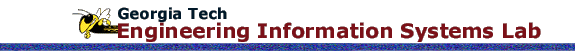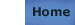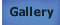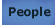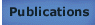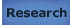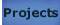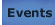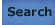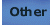# Performance Prediction of a Parallel Skyline Solver and its Implementation for Large Scale Structure Analysis

## Reference

Synn, S.Y.; Fulton, R.E. (1995) Computing Systems in Engineering: An International Journal, Vol. 6, No. 3, Pergamon Press Inc., Tarrytown, NY, USA, 275-284.

## Keywords

Parallel algorithms, Structural analysis, Performance, Computational complexity, Parallel processing systems, Data storage equipment, Computer simulation, Data communication systems, Computational methods, Large scale systems, Parallel skyline solver, Time complexity, Numerical operation, Computation stage, Recomputation stage

## Abstract

In this paper, we propose simplified formulas to predict the time complexity in a parallel skyline solver using two different memory schemes (Global Shard, Shared/Local memory) two machines (BBN, KSRI). Numerical operation counts and data communication costs are considered for the formulas. Based on these formulas, we developed a processor mapping algorithm to cover the initial computation stage and the recomputation stage of substructure analysis, and also compared the performances of parallel global approach and parallel substructure approach for practical structure models.